# Magic Eye Renderings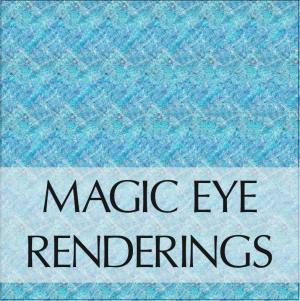Remember those Magic Eye books? I took some JPEG images from a trip to Hawaii and converted them to grayscale depth-mappings using Photoshop, and then used an algorithm (MagicEye.js) to generate “Magic Eye” images (technically single image random dot stereograms, or SIRDS).

This algorithm was published in a paper authored by by Harold W. Thimbleby, Stuart Inglis, and Ian H. Witten. The following code was translated from the C code that was featured in the article. http://www.cs.sfu.ca/CourseCentral/414/li/material/refs/SIRDS-Computer-94.pdf

The process works like this:

1. take a photograph that has obvious differences in depth, such as a person in front of a mountain.
2. Convert each major component of the image to a separate layer in Photoshop.
3. Using the basis that #000000 (black) is the furthest point in your image and #FFFFFF (white) is the closet, create a grayscale depth for each of your layers (or a gradient thereof), and finally merge and export the layers as a single grayscale image (PNG).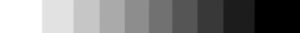4. Using the MagicEye algorithm, use this grayscale image to convert the final product as a “Magic Eye” image.

The Results: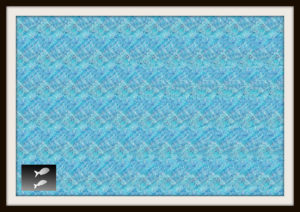Magic Eye – Tropical Fish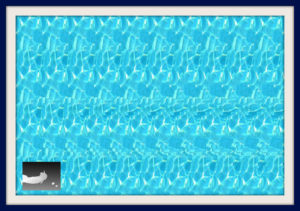Magic Eye – David Snorkeling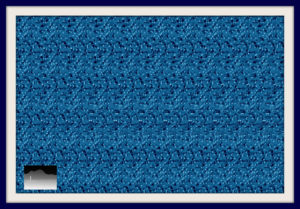Magic Eye – Hanalei Bay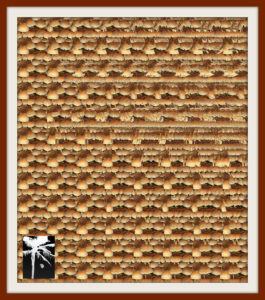Magic Eye – Palm Tree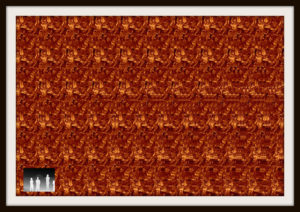Magic Eye – Waikiki Sunset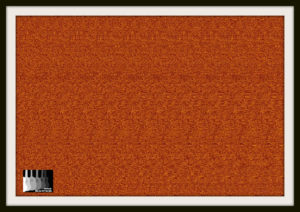Magic Eye – Ukulele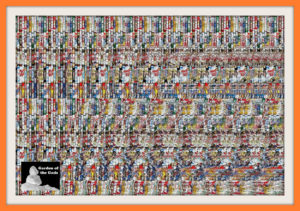Magic Eye – Garden of the Gods

Java Code Translated from C

``````generatePixelData: function (opts) {
var x, y, i, left, right, visible, t, zt, k, sep, z, pixelOffset, rgba,
width = opts.width,
height = opts.height,
depthMap = opts.depthMap,
numColors = opts.colors.length,
same, // points to a pixel to the right
dpi = 72, // assuming output of 72 dots per inch
eyeSep = Math.round(2.5 * dpi), // eye separation assumed to be 2.5 inches
mu = (1 / 3), // depth of field (fraction of viewing distance)
pixels = new Uint8ClampedArray(width * height * 4);

// for each row
for (y = 0; y < height; y++) {
// max image width (for Uint16Array) is 65536
same = new Uint16Array(width); // points to a pixel to the right

for (x = 0; x < width; x++) {
same[x] = x; // each pixel is initially linked with itself
}

// for each column
for (x = 0; x < width; x++) {

z = depthMap[y][x];

// stereo separation corresponding to z
sep = Math.round((1 - (mu * z)) * eyeSep / (2 - (mu * z)));

// x-values corresponding to left and right eyes
left = Math.round(x - ((sep + (sep & y & 1)) / 2));
right = left + sep;

if (0 <= left && right < width) {

// remove hidden surfaces
t = 1;
do {
zt = z + (2 * (2 - (mu * z)) * t / (mu * eyeSep));
visible = (depthMap[y][x-t] < zt) && (depthMap[y][x+t] < zt); // false if obscured
t++;
} while (visible && zt < 1);

if (visible) {
// record that left and right pixels are the same
for (k = same[left]; k !== left && k !== right; k = same[left]) {
if (k < right) {
left = k;
} else {
left = right;
right = k;
}
}
same[left] = right;
}
}
}

for (x = (width - 1); x >= 0; x--) {
pixelOffset = (y * width * 4) + (x * 4);
if (same[x] === x) {
// set random color
rgba = opts.colors[Math.floor(Math.random() * numColors)];
for (i = 0; i < 4; i++) {
pixels[pixelOffset + i] = rgba[i];
}
} else {
// constrained pixel, obey constraint
pixelOffset = (y * width * 4) + (x * 4);
for (i = 0; i < 4; i++) {
pixels[pixelOffset + i] = pixels[(y * width * 4) + (same[x] * 4) + i];
}
}
}
}

return pixels;
},``````

C Code

```<code>/* Algorithm for drawing an autostereogram */

#define round(X) (int)((X)+0.5) /* Often need to round rather than truncate */
#define DPI 72 /* Output device has 72 pixels per inch */
#define E round(2.5*DPI) /* Eye separation is assumed to be 2.5 in */
#define mu (1/3.0) /* Depth of field (fraction of viewing distance) */
#define separation(Z) round((1-mu*Z)*E/(2-mu*Z))
/* Stereo separation corresponding to position Z */
#define far separation(0) /* ... and corresponding to far plane, Z=0 */
#define maxX 256 /* Image and object are both maxX by maxY pixels */
#define maxY 256

void DrawAutoStereogram(float Z[][]) {
/* Object’s depth is Z[x][y] (between 0 and 1) */
int x, y; /* Coordinates of the current point */
for (y=0; y < maxY; y++) { /* Convert each scan line independently */
int pix[maxX]; /* Color of this pixel */
int same[maxX]; /* Points to a pixel to the right ... */
/* ... that is constrained to be this color */
int s; /* Stereo separation at this (x,y) point */
int left, right; /* X-values corresponding to left and right eyes */

for (x=0; x < maxX; x++)
same[x] = x; /* Each pixel is initially linked with itself */

for (x=0; x < maxX ; x++) {
s = separation(Z[x][y]);
left = x - s/2; /* Pixels at left and right ... */
right = left + s; /* ... must be the same ... */
if (0 <= left && right < maxX) { /* ... or must they? */
int visible; /* First, perform hidden-surface removal */
int t=1; /* We will check the points (x-t,y) and (x+t,y) */
float zt; /* Z-coord of ray at these two points */

do {
zt = Z[x][y] + 2*(2 - mu*Z[x][y])*t/(mu*E);
visible = Z[x-t][y]<zt && Z[x+t][y]<zt; /* False if obscured */
t++;
}

while (visible && zt < 1); /* Done hidden-surface removal ... */

if (visible) { /* ... so record the fact that pixels at */
int l = same[left]; /* ... left and right are the same */

while (l != left && l != right)

if (l < right) { /* But first, juggle the pointers ... */
left = l; /* ... until either same[left]=left */
l = same[left]; /* ... or same[left]=right */
}

else {
same[left] = right;
left = right;
l = same[left];
right = l;
}
same[left] = right; /* This is where we actually record it */
}
}
}
for (x=maxX-1 ; x>= 0 ; x--) {/* Now set the pixels on this scan line */
if (same[x] == x) pix[x] = random()&1; /* Free choice; do it randomly */
else pix[x] = pix[same[x]]; /* Constrained choice; obey constraint */
Set_Pixel(x, y, pix[x]);
}
}
DrawCircle(maxX/2-far/2, maxY*19/20); /* Draw convergence dots at far plane, */
DrawCircle(maxX/2+far/2, maxY*19/20); /* near the bottom of the screen */
}```